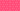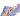PricingResources

# Multiple Linear Regression (MLR) Definition

Written by

While we can’t predict the future, there are statistical models that can help. One helpful calculation is the multiple linear regression model. It helps explore the relationship between cause and effect using a series of variables. Here’s how a multiple linear regression analysis can help you make more informed business decisions.

## What is multiple linear regression (MLR)?

Multiple linear regression, shortened to multiple regression or just MLR, is a technique used in statistics. Its purpose is to predict the likely outcome based on several variables, plotting the relationship between these multiple independent variables and single dependent variables.

The multiple linear regression model is based on a mathematical assumption that a linear relationship exists between both the independent and dependent variables. For this model to work, you also must assume that there’s no significant correlation between the multiple independent variables. This is because you’re measuring their impact on the dependent variable, not each other.

## Multiple linear regression formula

To perform a multiple linear regression analysis, you’ll need to plug a few basic values into the equation.

Here is the multiple linear regression formula written out:

• Y = predicted value of the dependent variable

• B0 = y-intercept or value of y with all parameters set to 0

• B1 X1 = regression coefficient of the first independent variable

• Bn Xn  = regression coefficient of the last independent variable

• E = model error or level of variation

It’s not the easiest calculation to do by hand, so in most cases you’ll need to use statistical software instead to see the results plotted on a graph for easier analysis.

## Multiple linear regression assumptions

To use this model, you need to take the following assumptions into account.

1. There is a linear relationship between each of the independent variables and the dependent variable. To test this, create scatterplots and verify a linear shape.

2. There aren’t high levels of correlation between any of the independent variables. If these variables are correlated both to each other and the dependent variable, this qualifies as multicollinearity and makes it more difficult to unpack the relationships.

3. The residuals should be normally distributed, according to multivariate normality. If there are any outlying patterns of distribution, this type of analysis may not be suitable.

## How to use the multiple linear regression model

Now that we’ve covered the multiple linear regression formula, you might be wondering how this helps you in the real world. Exploring the relationships between multiple variables and a single dependent variable can help you understand what’s driving price changes, market fluctuations, or sales figures. In most cases, these dependent variables are influenced by more than just one factor, which is why the multiple linear regression analysis is so useful.

By looking at a linear regression model, analysts can make predictions about the dependent variable based on data gleaned from multiple independent variables.

## Multiple linear regression example

What does this type of analysis look like in the workplace? Imagine that a financial analyst wants to understand more about how the wider market is impacting the price of an oil stock. In this multiple linear regression example, the independent variables could include things like the price of oil, interest rates, and oil future movements. The dependent variable would be the price of the oil stock.

By plugging all these variables into the equation, the analyst would then be able to see how fluctuations in the independent variables impact price movements of the dependent variable. The analyst would then know which variables to watch in order to more successfully predict future price movements, helping them decide when it’s time to buy or sell.

#### We can help

GoCardless helps you automate payment collection, cutting down on the amount of admin your team needs to deal with when chasing invoices. Find out how GoCardless can help you with ad hoc payments or recurring payments.## Interested in automating the way you get paid? GoCardless can help

Contact sales

Sales

Contact Sales

+1(415) 523-2279

Support

help@gocardless.com

+1 (628) 241-0044